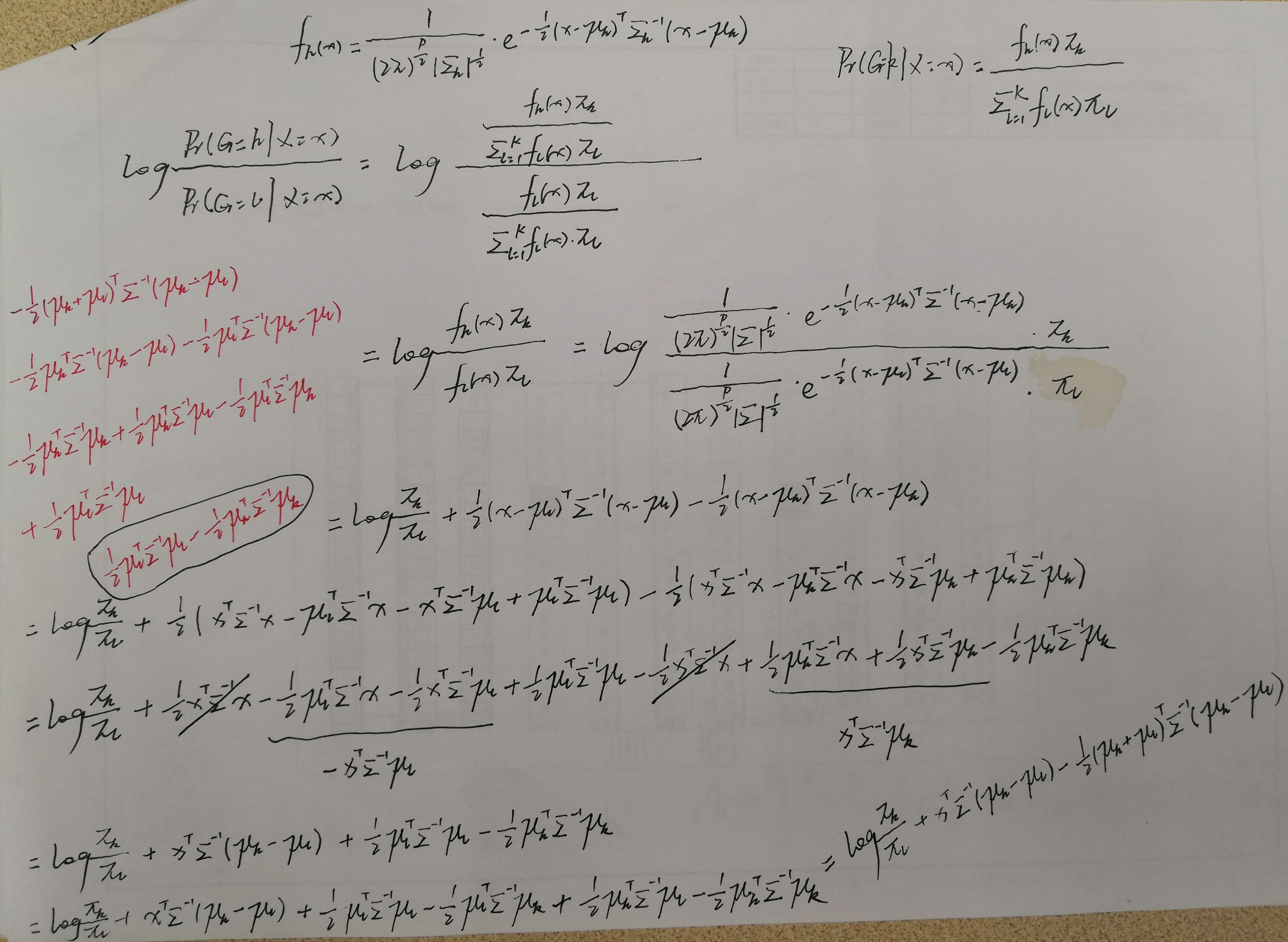﻿ Linear Discriminant Analysis

### Linear Discriminant AnalysisSuppose that we model each class density as multivariate Gaussian, in practice we do not know the parameters of the Gaussian distributions, and will need to

estimate them using our training data:

πk=Nk/N, where Nk is the number of class-k observations;

μkgi=k xi/Nk;